https://doi.org/10.5194/ms-12-529-2021
Special issue:
https://doi.org/10.5194/ms-12-529-2021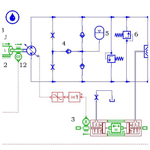# Performance analysis of an electro-hydrostatic actuator with high-pressure load sensing based on fuzzy PID

Hong Zhang, Lan Ding, Wentao Zhang, and Chenchen Li
Abstract

Electro-hydrostatic actuator (EHA) is an important form of power-by-wire (PBW) technology, which is widely used in aircraft because of the characteristics of small size, high power and light weight. However, the current traditional EHA with fixed pump displacement and variable motor speed (FPVM-EHA) has the problems of high energy consumption and heating of the motor under heavy load. An improved EHA with high-pressure load sensing (HPLS-EHA) is proposed in this paper, which can reduce the pump displacement under heavy load, so as to achieve reducing the torque and heating of the motor, solving the problem of high energy consumption of EHA system and improving the efficiency. The co-simulation model of the FPVM-EHA and the HPLS-EHA was established using the software programs AMESim and MATLAB, and then the simulation results are analysed. The simulation results show that the HPLS-EHA can reduce the torque and heat flow rate of the motor by 23.20 % and 41.02 % under the load of 20.2 MPa, and the fluctuation times and amplitude of output position are also reduced under varying loads at 5 s, but it will slightly reduce the position accuracy of the EHA system. In order to solve this problem, the fuzzy PID control strategy is adopted for the HPLS-EHA. The simulation results show that the position accuracy and response speed of the HPLS-EHA based on fuzzy PID are improved, the output position is improved from 8.93 to 9.25 mm, better than 9.19 mm of the FPVM-EHA, and it also maintains the advantages of low motor torque, heating and output position fluctuation.

Share
Dates
1 Introduction

Due to the low efficiency and complex structure of the traditional aircraft control system with the central hydraulic oil source, it has become the main constraint to improve the performance of the aircraft control system (Botten et al., 2000). With the development of multi-electric aircraft (MEA)/all-electric aircraft (AEA) technology, the application of power-by-wire (PBW) technology has been more widely used in aircraft (Mare and Fu, 2017). Electro-hydrostatic actuator (EHA) is an important form of PBW actuator, which is widely used in aircraft because of its advantages of large power, small size and light weight (Fu et al., 2015).

EHA is an independent hydraulic system using electric signal line, which is composed of a brushless DC motor, a high-pressure plunger axial pump, an accumulator, a hydraulic actuator and a control system. The working principle of the EHA is that the control system sends the electrical control signal to the servo motor according to the input command. The servo motor controls the rotational direction and speed of the pump after receiving the electrical signal. Then the pump delivers hydraulic oil to the piston end, creating a pressure difference on both sides of the piston so that the piston rod moves and drives the wings and rudder to work. According to different working conditions of the motor and pump, there are three control modes (Botten et al., 2000): constant pump displacement and variable motor speed, variable pump displacement and constant motor speed, and variable pump displacement and variable motor speed. At present, the FPVM-EHA is commonly used in aircraft, but for this kind of EHA, when the system bears a heavy load, the motor needs to output a large current to overcome the load torque, resulting in high heating and low efficiency of the system (Li et al., 2017).

The problems of high heating of motor and low efficiency of EHA system under heavy load have been the important factors hindering the further development of EHA. In order to solve this problem, based on the load-sensing technology, the FPVM-EHA is improved in this study, which is called the EHA with high-pressure load sensing (HPLS-EHA). According to the external load of the system, the control mode can be divided into two modes: constant pump displacement and variable motor speed under light load and variable pump displacement and variable motor speed under heavy load. The HPLS-EHA can reduce the displacement of the pump, the torque and heating of the motor and improve the efficiency of the system.

The structure of the whole paper is arranged as follows: Sect. 2 mainly presents the research on the principle of the high-pressure load-sensing mechanism, the establishment of simulation model and experimental verification of displacement regulator. Section 3 mainly focuses on the establishment and simulation of the co-simulation model of the HPLS-EHA and the FPVM-EHA. The simulation results show that the HPLS-EHA can reduce the motor torque and heating under heavy load and reduce the fluctuation under varying loads at 5 s, but it will reduce the position accuracy of the system. In order to solve the problem of low position accuracy of the system in Sect. 3, the main content of Sect. 4 is the establishment and simulation of the HPLS-EHA based on fuzzy PID. The simulation results show that the position accuracy of the HPLS-EHA based on fuzzy PID is better than the traditional FPVM-EHA. At the same time, the simulation results of motor torque, heating and output position fluctuation are consistent with Sect. 3. Section 5 summarizes the study work of this paper.

2 Principle and experimental verification of the displacement regulator with HPLS

## 2.1 Principle of displacement regulator with HPLS

The displacement regulator with HPLS is mainly composed of a motor, a hydraulic pump, a hydraulic cylinder, an electromagnetic relief valve and a displacement sensor, as shown in Fig. 1. The core component is the hydraulic cylinder with high-pressure spring in the right chamber, and the electromagnetic relief valve is used to simulate the external load. The working principle is that when the external load pressure is lower than the initial pressure of the right chamber of the hydraulic cylinder, the displacement regulator does not work, and the displacement maintains the maximum displacement. When the external load pressure is greater than the initial pressure of the right chamber, the displacement regulator works and the piston moves. The displacement sensor transmits the displacement signal of the piston to the hydraulic pump, so as to adjust the displacement of the hydraulic pump. In this study, when the external load pressure is 10 MPa, the displacement regulator starts to adjust until the external load reaches 21 MPa. At this time, the displacement signal received by the hydraulic pump is 0.75, and the minimum displacement of the variable pump is reached, which is 75 % of the maximum displacement.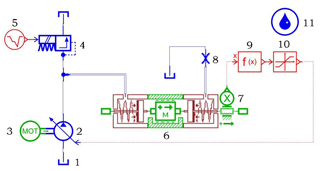Figure 1Simulation model of displacement regulator: (1) tank; (2) hydraulic pump; (3) motor; (4) electromagnetic relief valve; (5) pulse generator; (6) hydraulic cylinder; (7) displacement sensor; (8) throttle valve; (9) function generator; (10) signal limiter; (11) fluid properties.

According to the principle of displacement adjustment, the simulation software of AMESim is used to establish the model of displacement regulator. The simulation parameters are set as shown in Table 1.

The initial force of the spring at the right end is determined to be 10 MPa according to external load threshold, P0 is the initial pressure of the spring, S is the effective force area of the left end piston, R is the piston diameter, r is the rod diameter, and F0 is the initial force of the right end.

$\begin{array}{}\text{(1)}& {F}_{\mathrm{0}}={P}_{\mathrm{0}}S=\mathrm{10}×{\mathrm{10}}^{\mathrm{6}}×\frac{\mathit{\pi }}{\mathrm{4}}×\left({R}^{\mathrm{2}}-{r}^{\mathrm{2}}\right).\end{array}$

Substituting the simulation parameters in Table 1 into the Eq. (1), the F0 is calculated to be 2356.20 N.

K is the stiffness of the spring, which is determined according to the maximum value of the adjustment pressure. When the spring of right end is compressed to the end of the stroke, P1 is the load pressure at the left end, L is the stroke length of the spring, and the force F1 generated by the spring of right end at this time is

$\begin{array}{}\text{(2)}& & {F}_{\mathrm{1}}={P}_{\mathrm{1}}S=\mathrm{21}×{\mathrm{10}}^{\mathrm{6}}×\frac{\mathit{\pi }}{\mathrm{4}}×\left({R}^{\mathrm{2}}-{r}^{\mathrm{2}}\right)\text{(3)}& & K=\frac{{F}_{\mathrm{1}}-{F}_{\mathrm{0}}}{L}.\end{array}$

Substituting the simulation parameters in Table 1 into the Eq. (3), when the load pressure is 21 MPa, K is calculated to be 518.36 N mm−1.

Through the above calculation and analysis, all the simulation parameters of the displacement regulator can be obtained, which is very important for the correctness of the simulation model of the displacement regulator.

## 2.2 Experimental verification of displacement regulator with HPLS

To verify the mechanism of high-pressure load sensing, an EHA pump prototype was developed by the State Key Laboratory of Fluid Power and Mechatronic Systems, Zhejiang University (Xu et al., 2017; Chao et al., 2018), as shown in Fig. 2. Chao et al. (2018) verified the relationship between the load pressure and the pump displacement at different speeds, as shown in Fig. 3.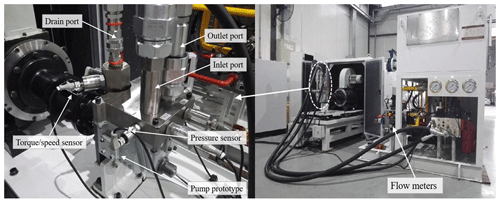Figure 2Experiments on the pump prototype. (a) Sensors installed in the pump prototype; (b) test rig for the pump prototype.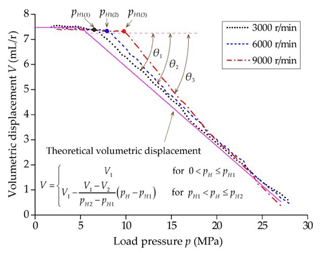Figure 3Comparison diagram of load pressure and volumetric displacement with different rotational speed.

It is clear from Fig. 3 that the displacement starts to drop with the increasing load pressure with the help of the load-sensing mechanism. At the same time, with the increase of the rotational speed, the displacement of the pump will decrease faster with the increase of the load pressure, which is reflected in the variation of the load threshold.

In order to verify the correctness of the simulation, set the simulation conditions the same as the experiment above: the motor speed is 3000, 6000 and 9000 rev min−1; the pump displacement is 7.5 mL rev−1; the overflow pressure rises uniformly from 0 to 21 MPa; the simulation time is 10 s; and the step size is 0.01 s. The overflow pressure and output flow curve with different rotational speed are shown in Fig. 4.

According to the simulation results in Fig. 4, when the overflow pressure is greater than 10 MPa, the pump displacement starts to decrease until the overflow pressure reaches 21 MPa, and the displacement is reduced to 75 % of the maximum displacement. It can be seen that the simulation data are consistent with the experimental data qualitatively by comparing Figs. 3 and 4, which verifies the correctness of the simulation model of displacement regulator and simulation method. However, because the simulation environment is ideal, different motor speed has no effect on the load threshold in the simulation.

3 Establishment and performance analysis of simulation model of the HPLS-EHA

## 3.1 Composition and working principle of the HPLS-EHA

The structural composition of the HPLS-EHA is shown in Fig. 5 (the solid line indicates the connection of the components, and the dashed line indicates the command and feedback signal). It is mainly composed of an EHA controller, a power drive unit, a brushless DC motor, a high-pressure plunger axial variable pump, a hydraulic circuit, an actuator cylinder, sensors, a displacement regulator and other components.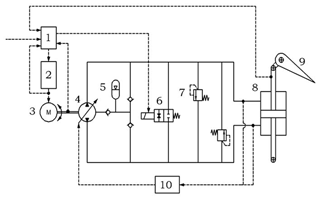Figure 5Schematic diagram of the HPLS-EHA: (1) EHA controller; (2) power drive unit; (3) brushless DC motor; (4) hydraulic pump; (5) energy accumulator; (6) damping bypass valve; (7) pressure relief valve; (8) actuator cylinder; (9) control surface; (10) displacement regulator.

The working principle is that the EHA controller receives input commands and various feedback signals. After corresponding calculations, the control signals of the motor are given through the motor drive unit. When the load pressure is less than 10 MPa, the control mode is constant pump displacement and variable motor speed, and the plunger pump outputs the hydraulic oil according to the motor speed to drive the cylinder to work. When the load pressure is greater than 10 MPa, the control mode is variable pump displacement and variable motor speed, and the plunger pump works according to the motor speed and pump displacement. The check valve simulates the leakage of the hydraulic pump. The energy accumulator is used as the system's pressurized oil tank, and it uses two check valves to replenish the system to maintain the minimum working pressure and prevent cavitation and air suction in the hydraulic circuit. The pressure relief valve is used to prevent the hydraulic system from generating excessive pressure, and the damping bypass valve opens when the system fails.

## 3.2 Establishment of simulation model

In order to improve the accuracy of modelling and the efficiency of simulation, this study uses the advantages of co-simulation with AMESim and MATLAB/Simulink. The hydraulic system is established by the AMESim platform, and the control system is established by the MATLAB platform (Ji et al., 2016).

According to the working principle of the HPLS-EHA system, the classical PID control of current loop and speed loop of motor and position loop of actuator cylinder is adopted. Firstly, the simulation model of the hydraulic system is established in AMESim, as shown in Fig. 6. Use the interface function to create the SimuCosim module, which is used to establish the connection relationship between AMESim and Simulink, and then use MATLAB/Simulink to complete the establishment of the control system model, as shown in Fig. 7.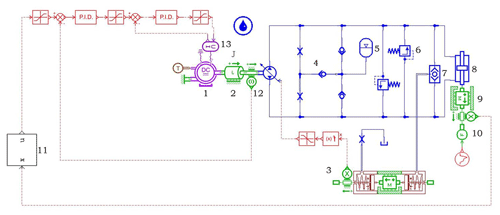Figure 6Simulation model of hydraulic system of the HPLS-EHA: (1) brushless DC motor; (2) moment of inertia module; (3) displacement regulator; (4) check valve; (5) energy accumulator; (6) pressure relief valve; (7) shuttle valve; (8) actuator cylinder; (9) load; (10) force generator; (11) SimuCosim module; (12) speed sensor; (13) current and voltage module.

In the modelling process, in order to facilitate the simulation analysis, the factors that have little effect on the simulation results are neglected, such as the setting of the bypass damping valve, the pressure drop between the hydraulic circuits, the damping and leakage of the cylinder, and linearization considerations of the brushless DC motor model, etc. The simulation parameters are shown in Table 2.

## 3.3 Simulation analysis of the HPLS-EHA

For the FPVM-EHA, the simulation model is similar to the HPLS-EHA: only the part of hydraulic pump is the quantitative pump, and the simulation model is no longer displayed. For the model of control system, the combination of PID control and fuzzy control is used in Fig. 7. There are two modes in the program of S-Function1: when M=1, the system uses PID control, and when M=2, the system uses fuzzy PID control. In this section, the simulation adopts PID control for the FPVM-EHA and HPLS-EHA.

Using the above simulation model, the parameters of Tables 1 and 2 are substituted into the model to analyse the performance under variable load. Set the simulation conditions as follows: the time is 10 s and the step is 0.01 s. At 0 s, an expected position signal with an amplitude of 10 mm is applied to the system, and a load of 10 kN is applied to the system. And a load of 30 kN is applied after 5 s. The comparison simulation results of the FPVM-EHA and the HPLS-EHA are shown in Fig. 8. Figure 8 shows the comparison of output torque of motor, Fig. 9 shows the comparison of heat flow rate of motor, and Fig. 10 shows the comparison of output position of actuator.

According to the load force received by the system, the system load pressure is 9 MPa for 0–5 s, and the system load pressure is 20.2 MPa for 5–10 s. The system load pressure is greater than the high-pressure load threshold of 10 MPa after 5 s; then the displacement of the HPLS-EHA begins to decrease. The following simulation analysis is based on the simulation results after 5 s.

It can be seen from Fig. 8 that the output motor's torque of the FPVM-EHA is 26.03 Nm, while the HPLS-EHA is 19.99 Nm, and the latter's torque is reduced by 23.20 %. Figure 9 shows that the motor's heat flow rate of the FPVM-EHA is 464.17 W, and the HPLS-EHA is 273.79 W. The latter has a 41.02 % reduction relative to the former.

From the above analysis, it can be concluded that the design of variable pump displacement under heavy load can effectively reduce the output torque and heat flow rate of the motor and significantly improve the working efficiency of the EHA.

It can be seen from Fig. 10 that the output position of the FPVM-EHA is 9.19 mm, while the HPLS-EHA is 8.93 mm. In the case of heavy load, although the torque and heat flow rate of the motor is reduced, the HPLS-EHA is poor in the position accuracy. However, when the load changes at 5 s, the position fluctuation of the HPLS-EHA is smaller, and the fluctuation times and amplitude are greatly reduced in the process of fluctuation to be stable, which is the advantage of displacement regulation to the system.

4 Simulation analysis of HPLS-EHA based on fuzzy PID

In order to solve the problem of low position accuracy described in Sect. 3, the fuzzy PID is used for the HPLS-EHA. In this section, fuzzy rules are set in MATLAB. Under the same simulation condition as Sect. 3, the position accuracy and response speed of the EHA system are simulated and analysed again.

## 4.1 Design of fuzzy PID controller

Fuzzy control has advantages of strong adaptability and of being robust, and there is no need to establish mathematical model, etc. It can realize the control when system parameters change at a large scale, but it cannot realize precision control. The traditional PID controller has the advantages of a simple algorithm, good stability and high reliability, but its application scope is small. Fuzzy PID combines the merit of fuzzy algorithm and PID algorithm, with deviation and deviation change rate as input, to adjust PID parameters; it realizes the precision control when system parameters change at a larger scale through adjusting the control parameters of PID in real time (Zheng, 2008; Ren and Qi, 2018; Ji et al., 2007). It is often used in systems with the problem of complex mathematical models, nonlinearities and time-varying uncertainties, which has the characteristics of fast response and good robustness; it can also meet the variable load (Yu et al., 2011; Song et al., 2018).

EHA is a complex nonlinear and time-varying uncertainty system. Aiming at the problem of low position accuracy of the HPLS-EHA under a heavy load, fuzzy PID control is adopted.

x and xt are set to the position deviation and deviation change rate, and ΔKp, ΔKi and ΔKd are the output parameters of the fuzzy controller. Then, the calculation formula of the output parameter of the fuzzy PID controller is as follows:

$\begin{array}{}\text{(4)}& {U}_{t}=k\left(\left({K}_{\mathrm{p}}+\mathrm{\Delta }{K}_{\mathrm{p}}\right)+\left({K}_{\mathrm{i}}+\mathrm{\Delta }{K}_{\mathrm{i}}\right)+\left({K}_{\mathrm{d}}+\mathrm{\Delta }{K}_{\mathrm{d}}\right)\right),\end{array}$

where k is the system proportional coefficient and Kp, Ki, and Kd are the coefficients of proportional, integral, and differential of the PID controller respectively.

The fuzzy controller can be designed by MATLAB software. The design process is mainly divided into three steps: fuzzification, fuzzy inference and clarification, which is carried out below.

A fuzzy controller of Mamdani type is established in MATLAB. The fuzzy subsets of the input quantities x and xt and the output quantities ΔKp, ΔKi, and ΔKd are all {NB, NM, NS, ZO, PS, PM, PB}. The input domain is (3, 3), and the output domain is (6, 6).

“And”, “Or”, “Implication”, “Aggregation” and “Defuzzification” are set to min, max, min, max and centroid. The curve of the membership function of the input and output is shown in Figs. 11 and 12 respectively.

According to the characteristics of the relationship between the input and output, 49 fuzzy rules are formulated and the fuzzy controller is established. At this time, the value of M in the program corresponding to S-Function1 in Fig. 5 is changed to 2, and fuzzy PID control is performed on the HPLS-EHA.

## 4.2 Simulation analysis of the HPLS-EHA based on fuzzy PID

It can be seen from Figs. 13 and 14 that the output position of the HPLS-EHA based on fuzzy PID is 9.68 mm at low pressure and 9.25 mm at high pressure, compared with the HPLS-EHA based on PID control. The output position is improved by 0.13 and 0.32 mm at low pressure and high pressure respectively, while the output position of the FPVM-EHA is 9.54 mm at low pressure and 9.19 mm at high pressure. Compared with the FPVM-EHA, the output position the HPLS-EHA based on fuzzy PID is improved by 0.14 and 0.06 mm at low pressure and high pressure respectively. At the same time, the response speed is improved; the fluctuation times and amplitude are also reduced when the load changes at 5 s.

According to the above simulation results under the simulation conditions in this research, the HPLS-EHA with fuzzy PID can ensure the position accuracy is better than the FPVM-EHA under the condition of reducing the motor heat flow rate by 41.02 %. At the same time, the response speed and fluctuation are also improved.

5 Conclusions

In order to solve the problem of high energy consumption and heating of the motor under heavy load, in this paper, a HPLS-EHA based on fuzzy PID is studied. The conclusions are as follows.

1. The high-pressure load sensing means that the displacement of the hydraulic pump is kept at a fixed displacement (maximum displacement) at low pressure, and the load-sensing function is only turned on under high pressure. Then the displacement of the pump is reduced accordingly.

2. Based on AMESim and MATLAB software, an EHA with the function of HPLS is established, which is called the HPLS-EHA. The system can reduce the torque and heat flow rate of the motor by reducing the displacement of the hydraulic pump under heavy load. In this study, the torque and heat flow rate of motor was reduced by 23.20 % and 41.02 % under the load of 20.2 MPa. Moreover, the fluctuation times and amplitude of output position are also reduced under the varying load at 5 s. But the disadvantage is that it will slightly reduce the position accuracy, which is 0.26 mm lower than the output position of the FPVM-EHA.

3. The HPLS-EHA based on fuzzy PID can improve the position accuracy of the actuator from 8.93 to 9.25 mm, which is 0.06 mm higher than the output position 9.19 mm of the FPVM-EHA while reducing the torque and heat flow rate of motor by 23.20 % and 41.02 %, respectively. At the same time, this improves the response speed and fluctuation.

In summary, the HPLS-EHA based on the fuzzy PID is superior to the FPVM-EHA in terms of torque and heating of motor, position accuracy, response speed and fluctuation of system. This research has made some efforts for the heating of the motor, energy conservation of the aircraft and the performance upgrade of the flight control system.

Data availability

All the data used in this paper can be obtained from the corresponding author upon request.

Author contributions

HZ provided important theoretical and academic guidance in the research; LD established co-simulation model of the HPLS-EHA and the FPVM-EHA; WTZ carried out simulation analysis of the model; CCL analysed and summarized the simulation results; LD, WTZ and CCL provided help for the modification of the picture and format of the article.

Competing interests

The authors declare that they have no conflict of interest.

Special issue statement

Acknowledgements

The authors acknowledge the financial supported by the National Natural Science Foundation of China.

Financial support

This work was supported by the National Nature Science Foundation of China (grant no. 51975092).

Review statement

This paper was edited by Bo Li and reviewed by Xuejing Liu and two anonymous referees.

References

Botten, S. L., Whitley, C. R., and King, A. D.: Flight control actuation technology for next-generation all-electric aircraft, Technology Review Journal, 8, 55–68, 2000.

Chao, Q., Zhang, J., Xu, B., Shang, Y., Jiao, Z., and Li, Z.: Load-Sensing Pump Design to Reduce Heat Generation of Electro-Hydrostatic Actuator Systems, Energies, 11, 2266, https://doi.org/10.3390/en11092266, 2018.

Fu, Y.-L., Shao, Y.-B., and Qi, H.: Integrated Electro-hydrostatic Actuator System: Theory and Technology, Chinese Hydraulics and Pneumatics, 0, 1–8, https://doi.org/10.11832/j.issn.1000-4858.2015.05.001, 2015.

Gao, B., Fu, Y.-L., Pei, Z.-C., and Ma, J.-M.: Research on Dual-Variable Integrated Electro-Hydrostatic Actuator, Chinese J. Aeronaut., 19, 77–82, https://doi.org/10.1016/S1000-9361(11)60271-9, 2006.

Huang, L., Yu, T., Jiao, Z., and Li, Y.: Active Load-Sensitive Electro-Hydrostatic Actuator for More Electric Aircraft, Appl. Sci., 10, 6978, https://doi.org/10.3390/app10196978, 2020.

Huang, Z.-P., Lou, H., and Wang, J.-S.: Design And Simulation Analysis of a New Type EHA Based on Load-sensing Technology, Hydraulics Pneumatics and Seals, 32, 31–33, 2012.

Ji, T.-L., Hai-tao, Q., and Ya-ting, T.: Analysis of Sliding-mode Control for EHA Based on AMESim and MATLAB Co-Simulation, Chinese Hydraulics and Pneumatics, 0, 19–24, https://doi.org/10.11832/j.issn.1000-4858.2016.03.004, 2016.

Ji, Y., Xiaoye, Q., and Zhongcai, P.: Pump and valve combined scheme of EHA based on variable pressure, The Fifth International Symposium on Fluid Power Transmission and Control, Chinese Mechanical Engineering Society, Beijing, 855–858, 2007.

Jiang, J.-H., Jun-long, L., Qiao, L., Huan-ling, Z., and Jin-bao, Z.: Design and Simulation of Electro?hydrostatic Actuation System Controlled by Variable Pump and Variable Motor Composite for Rocket Rudder, Chinese Hydraulics and Pneumatics, 0, 11–15, https://doi.org/10.11832/j.issn.1000-4858.2015.01.003, 2015.

Li, S., Shang, Y., Wu, S., Zhou, Y., and Jiao, Z.: Investigation the load matching of direct pressure valve controlled variable mechanism of axial variable piston pump, 2017 IEEE International Conference on Cybernetics and Intelligent Systems (CIS) and IEEE Conference on Robotics, Automation and Mechatronics (RAM), 19–21 November 2017, 434–438, https://doi.org/10.1109/ICCIS.2017.8274815, 2017.

Mare, J.-C. and Fu, J.: Review on signal-by-wire and power-by-wire actuation for more electric aircraft, Chinese J. Aeronaut., 30, 857–870, https://doi.org/10.1016/j.cja.2017.03.013, 2017.

Ren, B. and Qi, X.-Y.: Electric Hydrostatic Actuator Position Control System Based on Fuzzy PID, Automation and Instrumentation, 33, 2018.

Song, C., Cao, A., and Wen, J.: Design of Adaptive Fuzzy PID Controller and MATLAB Simulation, Modern Manufacturing Technology and Equipment, 203–205, 2018.

Xu, B., Chao, Q., Zhang, J., and Chen, Y.: Effects of the dimensional and geometrical errors on the cylinder block tilt of a high-speed EHA pump, Meccanica, 52, 2449–2469, https://doi.org/10.1007/s11012-016-0590-0, 2017.

Yu, L.-K., Jian-ming, Z., Qi-long, Y., Ji-ming, X., and Yan, L.: Fuzzy PID control for direct drive electro-hydraulic position servo system, 2011 International Conference on Consumer Electronics, Communications and Networks (CECNet), 16–18 April 2011, 370–373, https://doi.org/10.1109/CECNET.2011.5768670, 2011.

Zheng, Q.: Xinmin, Shi Fuzzy Control and Its MATLAB Simulation, Tsinghua University Press, Beijing Jiaotong University Press, Beijing, 2008.